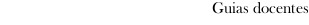Guia docente 2019_20Escuela de Ingeniería Industrial
 Degree in Mechanical EngineeringSubjectsMathematics: Calculus II and differential equationsContents
 Topic Sub-topic Integral calculus in several variables. The double integral on rectangles. Cavalieris Principle. Reduction to iterate integrals. Double integral on elementary regions. Properties. Fubinis theorem. The change of variables theorem. The particular case of polar coordinates. Triple integrals on a box and elementary regions. Fubinis theorem. The change of variables theorem. Particular cases: cylindrical and spherical coordinates. Geometric and physical applications of multiple integration: computation of volumes, mass centers and inertia momentums. Vector calculus. Curves in the plane and in three-dimensional space. Arc length. Change of parameter. Line or trajectory integrals with respect to the arc length of scalar fields. Line integral or circulation of vector fields. Properties. Fundamental theorem of line integrals. Greens theorem on the plane. Regular surfaces. Tangent plane. Normal vector. Area of a Surface. Surface integral of scalar fields. Flux or surface integral of vector fields. Divergence and curl operators. Characterization of conservative fields. Stokes theorem. Gauss theorem. Differential equations. Ordinary differential equations. Concept of solution of an ordinary differential equation. Theorems of existence and uniqueness for problems with initial conditions. Methods of solution of first order differential equations: equations in separable variables, equations reducible to separable variables, homogeneuous equations, linear and linear reduced equations. Exact differential equations. Integrating factors. Differential equation of a uniparametric family of plane curves. Orthogonal trajectories. Linear differential equations of order two and greater. Initial condition problems. Fundamental sets. Method of variation of parameters . Method of undetermined coefficients. Order reduction. Eulers equation. Systems of linear differential equations. Numerical methods for initial value problems. Introduction to numerical methods. Eulers and improved Eulers method. Runge-Kuttas fourth order method.
 Universidade de Vigo            | Rectorado | Campus Universitario | C.P. 36.310 Vigo (Pontevedra) | España | Tlf: +34 986 812 000